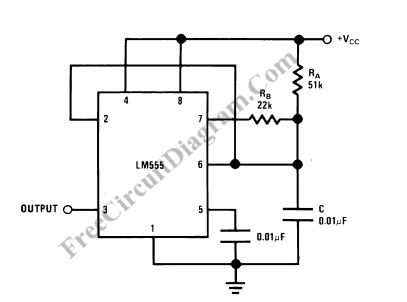# 555 IC Oscillator with 50% Duty CycleA square wave oscillator using 555 IC can be configured to give symmetric oscillation (50% duty cycle). Other circuit uses diodes to split the charging and the discharging paths through different resistors, but here we need no such diodes. Here is the schematic diagram:The time period for the output high is the same as regular configuration,

t1 = 0.693 RA C,

but for the output low, the period is

t2=[(RA.RB/(RA+RB)].C.Ln[(RB-2RA)/(2RB-RA)]

Thus the oscillator frequency is  equal to 1/(t1+t2).

Please remember this oscillator circuit will not oscillate if RB is greater than 1/2 RA because the junction of RA and RB cannot bring pin 2 down to 1/3 VCC and trigger the lower comparator. [Circuit’s schematic diagram source:  National Semiconductor Application Notes]